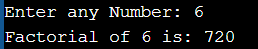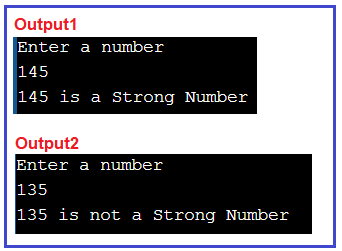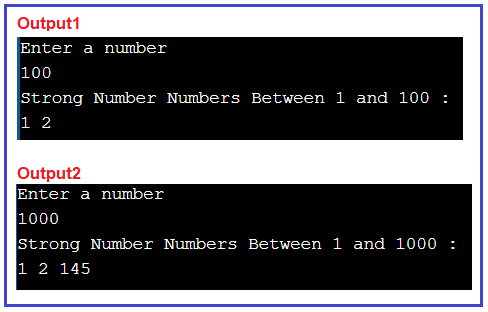# Strong Number in C#

## Strong Number Program in C# with Examples

In this article, I am going to discuss How to Implement the Strong Number Program in C# with Examples. Please read our previous article where we discussed the Buzz Number Program in C#. Here, in this article, first, we will learn what is a Strong Number and then we will see how to implement the Strong Number Program in C#. And finally, we will see how to print all the Strong numbers between a range of numbers like between 1 to 100 or 100 to 1000, etc.

##### What is a Strong Number?

A Strong number is a special number whose sum of the factorial of each digit is equal to the original number.

Examples:
Input: 145
Output: Yes, it is a strong number
Explanation: 1! + 4! + 5! = 145

Input: 124
Output: No, it is not a strong number
Explanation: 1! + 2! + 4! = 27 which is not equal to n i.e., 124

First of all, we have to know what is Factorial number. how it works. What is the basic method to solve this problem?

Factorial number: Factorial of n is the product of all positive descending integers.
For example
1) 5! = 5*4*3*2*1 = 120
2) 3! = 3*2*1 = 6

##### Factorial of Number in C#
```using System;
public class FactorialExample
{
public static void Main ()
{
int i, fact = 1, n;
Console.Write ("Enter any Number: ");
for (i = 1; i <= n; i++)
{
fact = fact * i;
}
Console.Write ("Factorial of " + n + " is: " + fact);
}
}
```
###### Output:More Details: –

After understanding how to calculate the factorial of a number let’s come to our main question which is a strong number.

##### How to Implement Strong Number Program in C#?

Let us understand how to check whether a given number is a strong number or not in C#. The following are the steps that we need to follow to solve this problem.

1. First, we take the input Number.
2. Then we separate the individual number of digits.
3. Then we calculate the factorial of the individual number of digits.
4. After calculating factorial, we will sum the factorial of the individual digit.
##### Example: Strong Number in C#

The following sample code shows how to implement the strong number program in C#.

```using System;
public class StrongNumberProgram
{
public static void Main ()
{
int number = 0, sum = 0;
Console.WriteLine ("Enter a number");

int quot = number;
int remainder;

while (quot != 0)
{
remainder = quot % 10;
int fact = CalculateFactorial(remainder);
quot = quot / 10;
sum = sum + fact;
}
if(sum == number)
{
Console.WriteLine (number + " is a Strong Number");
}
else
{
Console.WriteLine (number + " is not a Strong Number");
}
}

//Calulate Factorial of a number
public static int CalculateFactorial(int number)
{
int fact = 1;
for (int i = 1; i <= number; i++)
{
fact = fact * i;
}
return fact;
}
}
```
###### Output:TIME COMPLEXITY: Time Complexity: O(N)

##### Print all strong number from 1 to N (input value from user)

Input: Number = 100
Output: 1 2
Explanation: Only 1 and 2 are the strong numbers from 1 to 100 because
1! = 1, and
2! = 2

Input: N = 1000
Output: 1 2 145
Explanation: Only 1, 2 and 145 are the strong numbers from 1 to 1000 because
1! = 1,
2! = 2, and
(1! + 4! + 5!) = 145

The idea is to iterate from [1, Number] and check if any number between that range is a strong number or not. If yes then print that number, else check for the next number.

##### C# Program to Print all Strong Numbers Between 1 and N

The following C# Program will allow the user to input a number and then it will print all the strong numbers between 1 and that input number.

```using System;
public class StrongNumberProgram
{
public static void Main ()
{
int number = 0;
Console.WriteLine ("Enter a number");

int remainder;
Console.WriteLine ("Strong Number Numbers Between 1 and "+ number + " : ");
for(int i = 1; i<= number; i++)
{
int quot = i, sum = 0;
while (quot != 0)
{
remainder = quot % 10;
int fact = CalculateFactorial(remainder);
quot = quot / 10;
sum = sum + fact;
}
if(sum == i)
{
Console.Write(i + " ");
}
}
}

//Calulate Factorial of a number
public static int CalculateFactorial(int number)
{
int fact = 1;
for (int i = 1; i <= number; i++)
{
fact = fact * i;
}
return fact;
}
}
```
###### Output:In the next article, I am going to discuss the Kaprekar Number Program in C# with Examples. Here, in this article, I try to explain How to Implement the Strong Number Program in C# with Examples and I hope you enjoy this article.